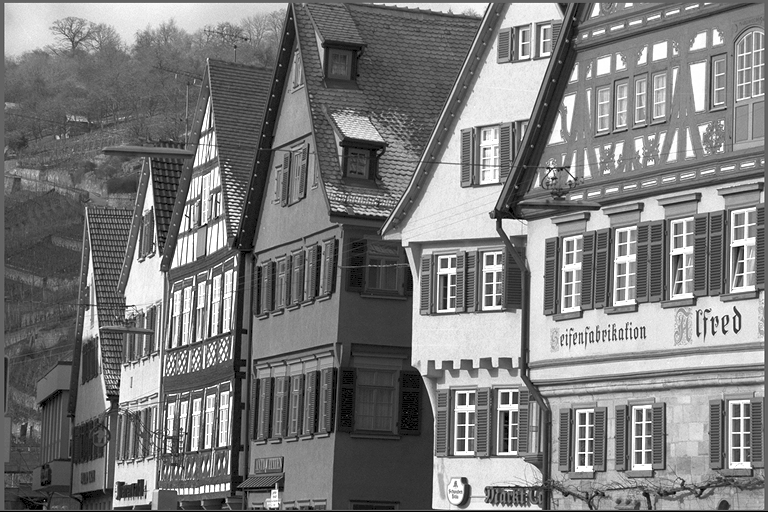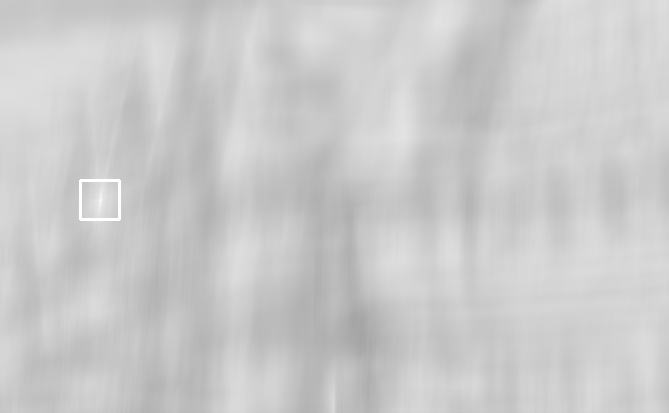## VPI - Vision Programming Interface

#### 2.2 Release

Template Matching

# Overview

Template matching is a method for finding the best matched location of a template image inside a larger image. We use the normalized cross-corrleation to compute the best match.

The following is an example showing a source image on the left with the template image in the middle. The matched score of output image is shown in the right.

Input Template Output score converted to U8# Implementation

The algorithm computes the normalized cross correlation (score) for every possible location of the template inside the source image. The location with the highest score is chosen as the best matching location between source and template image.

The cross correlation is calculated as follows:

\begin{align*} R(x, y) &= \frac { \sum_{x\prime, y\prime}( (T(x\prime, y\prime) - I(x+x\prime, y+y\prime)) \cdot M(x\prime, y\prime))^2 } { \sqrt{ \sum_{x\prime, y\prime} (T(x\prime, y\prime) \cdot M(x\prime, y\prime))^2 \cdot \sum_{x\prime, y\prime} (I(x+x\prime, y+y\prime) \cdot M(x\prime, y\prime))^2} } \end{align*}

where:
$$T(x\prime, y\prime)$$ refers to the template image.
$$I(x+x\prime, y+y\prime)$$ refers to the source image.
$$M(x\prime, y\prime)$$ refers to the mask.
$$x\prime$$ and $$y\prime$$ are the pixel coordinates inside the template image.

If the source image has a resolution W $$\times$$ H and template image w $$\times$$ h, then the output image has a resolution (W - w + 1) $$\times$$ (H - h + 1). If the template exceeds a size of 400 pixels, the convolution will be computed in Fourier space, otherwise in original space.

# C API functions

For list of limitations, constraints and backends that implements the algorithm, consult reference documentation of the following functions:

Function Description
vpiTemplateMatchingSetSourceImage Set the source image.
vpiTemplateMatchingSetTemplateImage Set the template image.
vpiSubmitTemplateMatching Runs the template matching algorithm with provided template.

# Usage

Language:
1. Import VPI module
import vpi
2. Match the template image in the input image by using CUDA backend. It's using the normalized cross-correlation.
with vpi.Backend.CUDA:
output = vpi.templateMatching(input, templ)
1. Initialization phase
1. Include the header that defines the template matching function.
Declares functions that implement the template matching algorithm.
2. Define the input image object.
VPIImage input = /*...*/;
struct VPIImageImpl * VPIImage
A handle to an image.
Definition: Types.h:256
3. Define the template image object.
VPIImage templ = /*...*/;
4. Create an output image with the required size and format.
int32_t srcW, srcH;
vpiImageGetSize(input, &srcW, &srcH);
int32_t templW, templH;
vpiImageGetSize(templ, &templW, &templH);
VPIImage output;
vpiImageCreate(srcW - templW + 1, srcH - templH + 1, VPI_IMAGE_FORMAT_F32, 0, &output);
#define VPI_IMAGE_FORMAT_F32
Single plane with one 32-bit floating point channel.
Definition: ImageFormat.h:130
VPIStatus vpiImageCreate(int32_t width, int32_t height, VPIImageFormat fmt, uint64_t flags, VPIImage *img)
Create an empty image instance with the specified flags.
VPIStatus vpiImageGetSize(VPIImage img, int32_t *width, int32_t *height)
Get the image dimensions in pixels.
5. Create the stream where the algorithm will be submitted for execution.
VPIStream stream;
vpiStreamCreate(0, &stream);
struct VPIStreamImpl * VPIStream
A handle to a stream.
Definition: Types.h:250
VPIStatus vpiStreamCreate(uint64_t flags, VPIStream *stream)
Create a stream instance.
6. Create the payload that will contain all temporary buffers needed for processing. It'll be created on the CUDA backend.
A handle to an algorithm payload.
Definition: Types.h:268
@ VPI_BACKEND_CUDA
CUDA backend.
Definition: Types.h:93
2. Processing phase
1. Set the input image.
Set the source image.
2. Set the template image.
Set the template image.
3. Submit the algorithm to the stream along with all parameters. It'll be executed by the CUDA algorithm.
Runs the template matching algorithm with provided template.
@ VPI_TEMPLATE_MATCHING_NCC
Normalized cross correlation.
4. Optionally, wait until the processing is done.
vpiStreamSync(stream);
VPIStatus vpiStreamSync(VPIStream stream)
Blocks the calling thread until all submitted commands in this stream queue are done (queue is empty)...
3. Cleanup phase
1. Free resources held by the stream and the input and output images.
vpiImageDestroy(output);
void vpiImageDestroy(VPIImage img)
Destroy an image instance.
void vpiStreamDestroy(VPIStream stream)
Destroy a stream instance and deallocate all HW resources.

Consult the Template Matching for a complete example.

For more information, see Template Matching Algorithm in the "C API Reference" section of VPI - Vision Programming Interface.

# Performance

Performance benchmarks will be added at a later time.

## References

1. Briechle, K., & Hanebeck, U. D. (2001, March). Template matching using fast normalized cross correlation. In Optical Pattern Recognition XII (Vol. 4387, pp. 95-102). SPIE.
2. Chen, C. S., Huang, C. L., Yeh, C. W., & Chang, W. C. (2016). An accelerating CPU based correlation-based image alignment for real-time automatic optical inspection. Computers & Electrical Engineering, 49, 207-220.